# Beyond Bar and Box Plots

Hands–on code to create and style chart alternatives with ggplot2

Cédric Scherer https://cedricscherer.com
2021-11-04

The slides are available here.
Alternatively, you can watch the recording on YouTube.

## Packages

``````library(tidyverse)     ## data wrangling + ggplot2
library(rcartocolor)   ## Carto palettes
library(ggforce)       ## sina plots
library(ggdist)        ## halfeye plots
library(ggridges)      ## ridgeline plots
library(ggbeeswarm)    ## beeswarm plots
library(gghalves)      ## off-set jitter
library(systemfonts)   ## custom fonts
``````

## Import Data

We are using a synthetic data set consisting of four groups with differing sample sizes and diustributions of some values.

``````url <- "https://raw.githubusercontent.com/z3tt/DataViz-Teaching/master/data/weissgerber-data.csv"
``````

The data was generated with the code provided in the flipbook by Nico Riedel, Robert Schulz, and Tracey Weissgerber.

## ggplot2 Setup

To use a custom font, one needs to install the .ttf or .otf font files on the local machine. Here I am using the well-known Roboto typefaces that are available via GoogleFonts:

(In case you can’t install the fonts, you might see some warnings that you can ignore. In case it throws an error, remove all arguments related to fonts, i.e. `family` and `base_family` arguments.)

``````## general theme
theme_set(theme_void(base_family = "Roboto"))

theme_update(
axis.text.x = element_text(color = "black", face = "bold", size = 26,
margin = margin(t = 6)),
axis.text.y = element_text(color = "black", size = 22, hjust = 1,
margin = margin(r = 6), family = "Roboto Mono"),
axis.line.x = element_line(color = "black", size = 1),
panel.grid.major.y = element_line(color = "grey90", size = .6),
plot.background = element_rect(fill = "white", color = "white"),
plot.margin = margin(rep(20, 4))
)

## theme for horizontal charts
theme_flip <-
theme(
axis.text.x = element_text(face = "plain", family = "Roboto Mono", size = 22),
axis.text.y = element_text(face = "bold", family = "Roboto", size = 26),
panel.grid.major.x = element_line(color = "grey90", size = .6),
panel.grid.major.y = element_blank(),
legend.position = "top",
legend.text = element_text(family = "Roboto Mono", size = 18),
legend.title = element_text(face = "bold", size = 18, margin = margin(b = 25))
)

## custom colors
my_pal <- rcartocolor::carto_pal(n = 8, name = "Bold")[c(1, 3, 7, 2)]
``````

## Summary Plots

### Barplot

``````ggplot(data, aes(x = group, y = value, fill = group)) +
geom_bar(stat = "summary", width = .8) +
scale_y_continuous(expand = c(0, 0), breaks = 0:4) +
scale_fill_manual(values = my_pal, guide = "none")
``````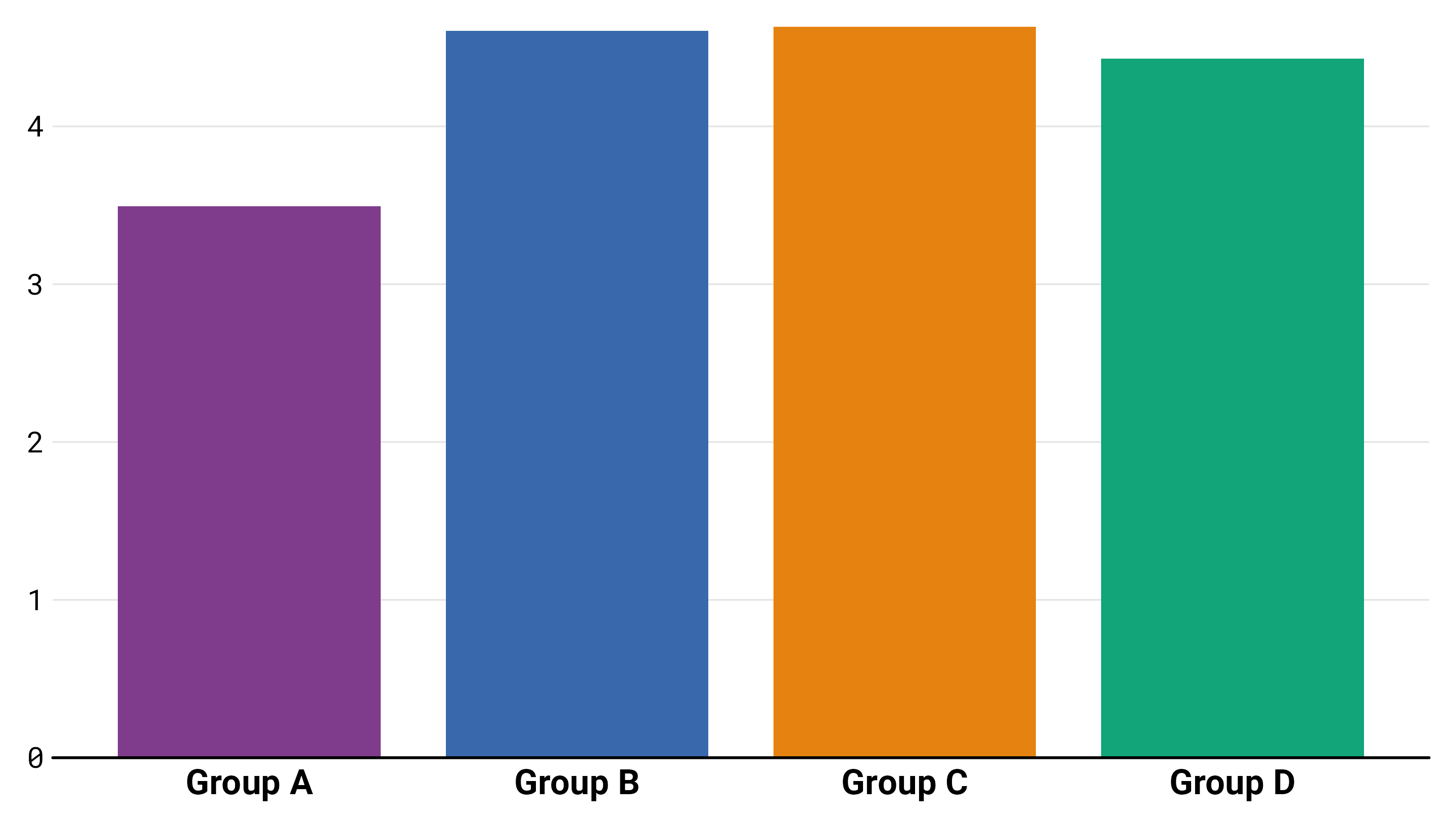Most data are not as clean as bar plots make them seem, and since bar plots reveal very little about the distribution of the data, this kind of visualization can be misleading. A further issue is that of the bar itself, which implies that the base of the y-axis is meaningful, which is not necessarily the case. ~ #barbarplot intiative

``````ggplot(data, aes(x = group, fill = group)) +
geom_bar(width = .8) +
scale_y_continuous(expand = c(0, 0)) +
scale_fill_manual(values = my_pal, guide = "none")
``````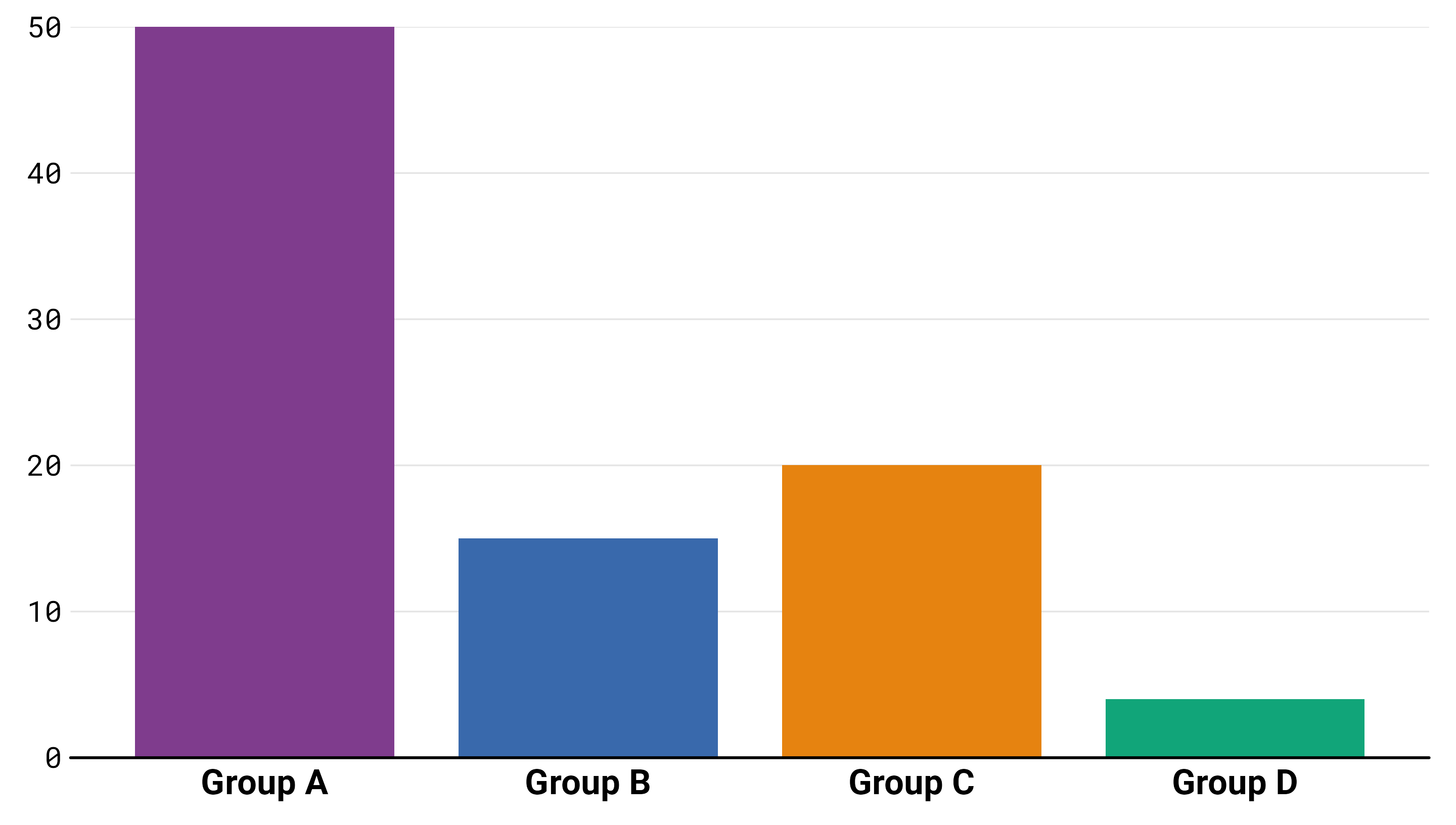### Dynamite Plot

Dynamite plots are used to compare measurements from two or more groups: cases and controls, for example. In a two group comparison, the plots are graphical representations of a grand total of 4 numbers, regardless of the sample size. The four numbers are the average and the standard error (or the standard deviation, it’s not always clear) for each group. ~ Rafael Irizarry, “Dynamite Plots Must Die”

``````ggplot(data, aes(x = group, y = value, color = group, fill = group)) +
stat_summary(
geom = "errorbar",
fun.max = function(x) mean(x) + sd(x),
fun.min = function(x) mean(x) - sd(x),
width = .3, size = 1.2
) +
geom_bar(stat = "summary", width = .8, size = .8) +
scale_y_continuous(expand = c(0, 0), breaks = 1:9) +
scale_fill_manual(values = my_pal, guide = "none") +
scale_color_manual(values = my_pal, guide = "none")
``````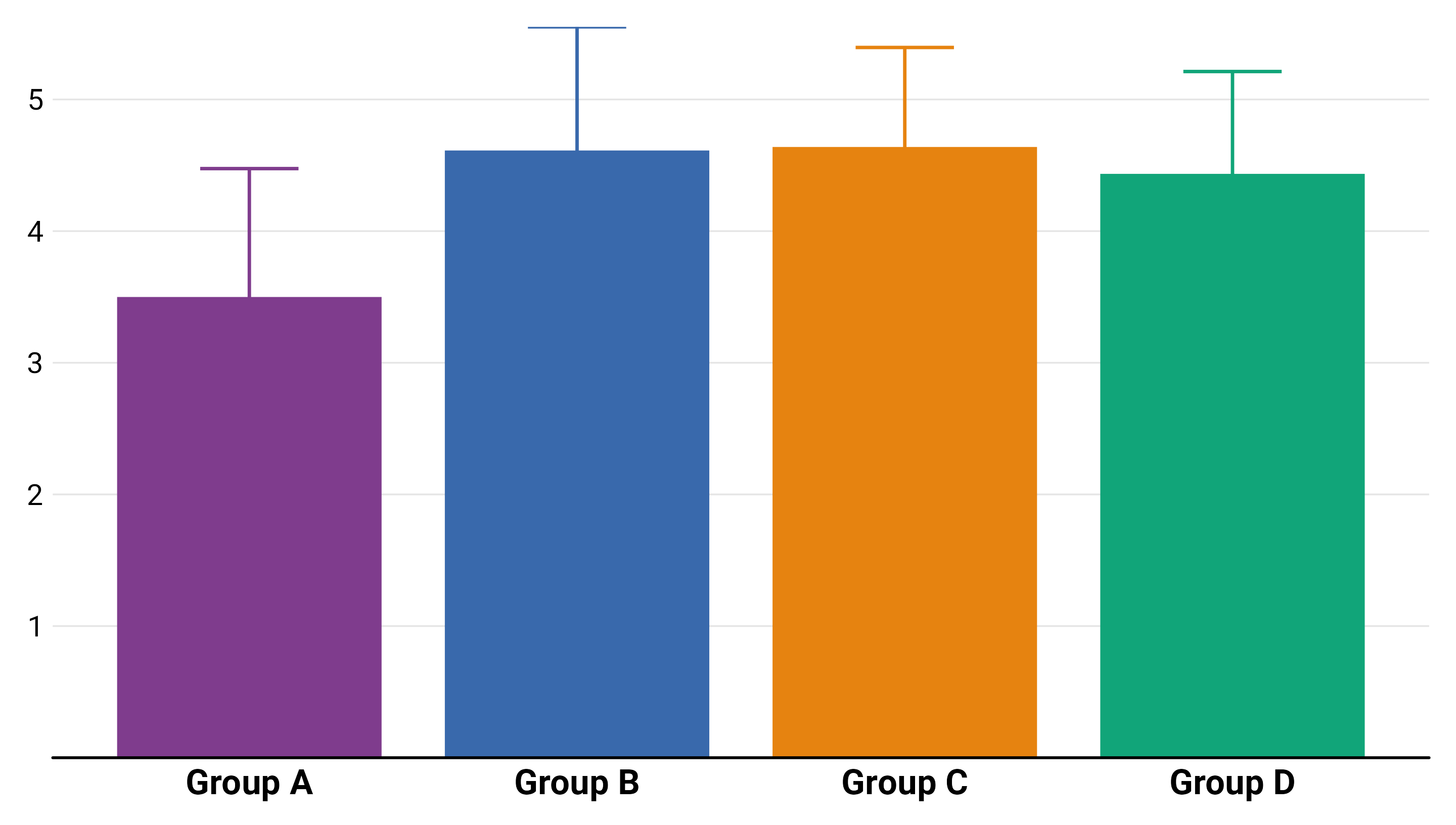### Boxplot

``````g <- ggplot(data, aes(x = group, y = value, color = group, fill = group)) +
scale_y_continuous(breaks = 1:9) +
scale_color_manual(values = my_pal, guide = "none") +
scale_fill_manual(values = my_pal, guide = "none")
``````
``````g +
geom_boxplot(alpha = .5, size = 1.5, outlier.size = 5)
``````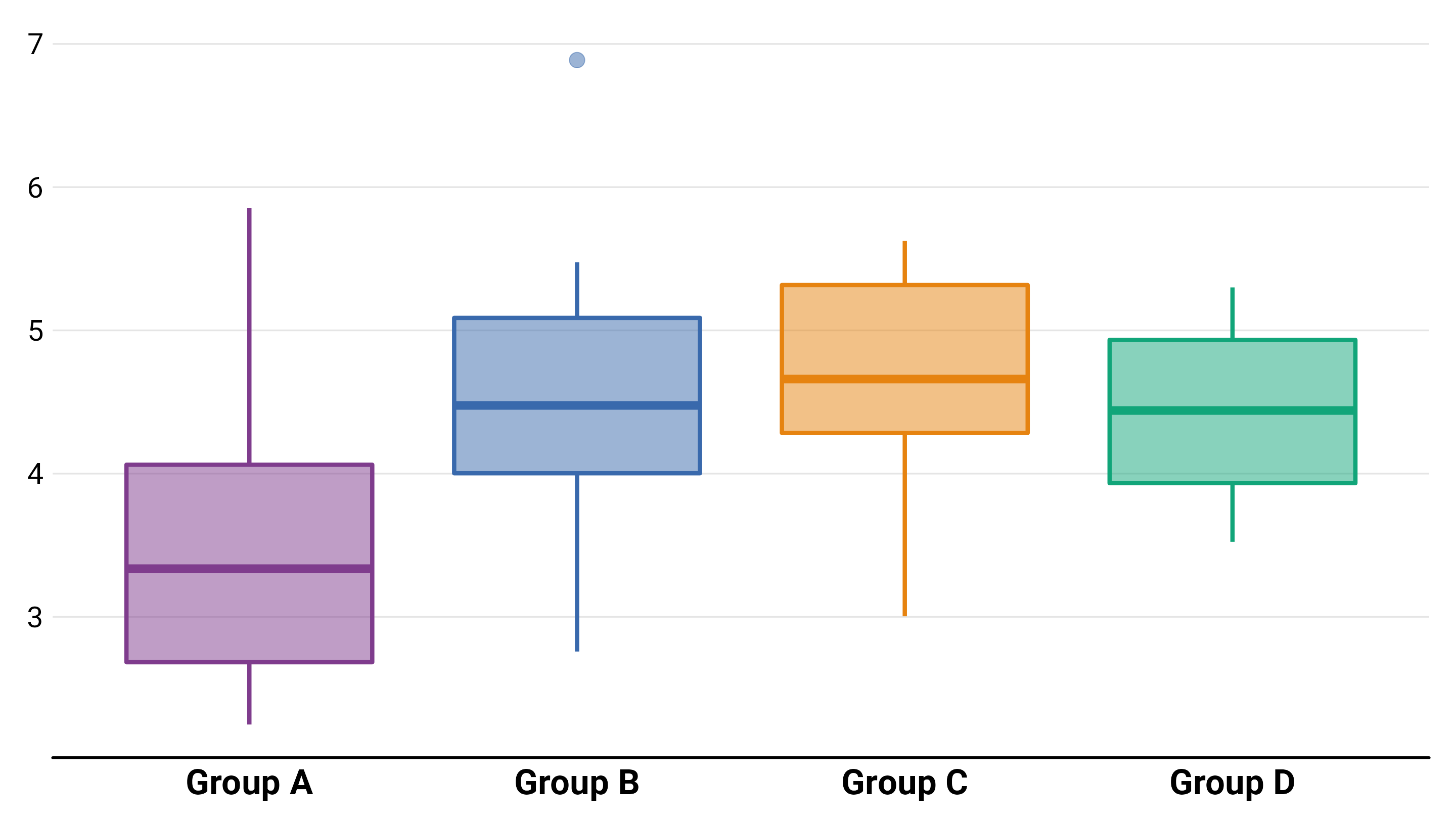``````g +
geom_boxplot(
aes(fill = group, fill = after_scale(colorspace::lighten(fill, .5))),
size = 1.5, outlier.size = 5
)
``````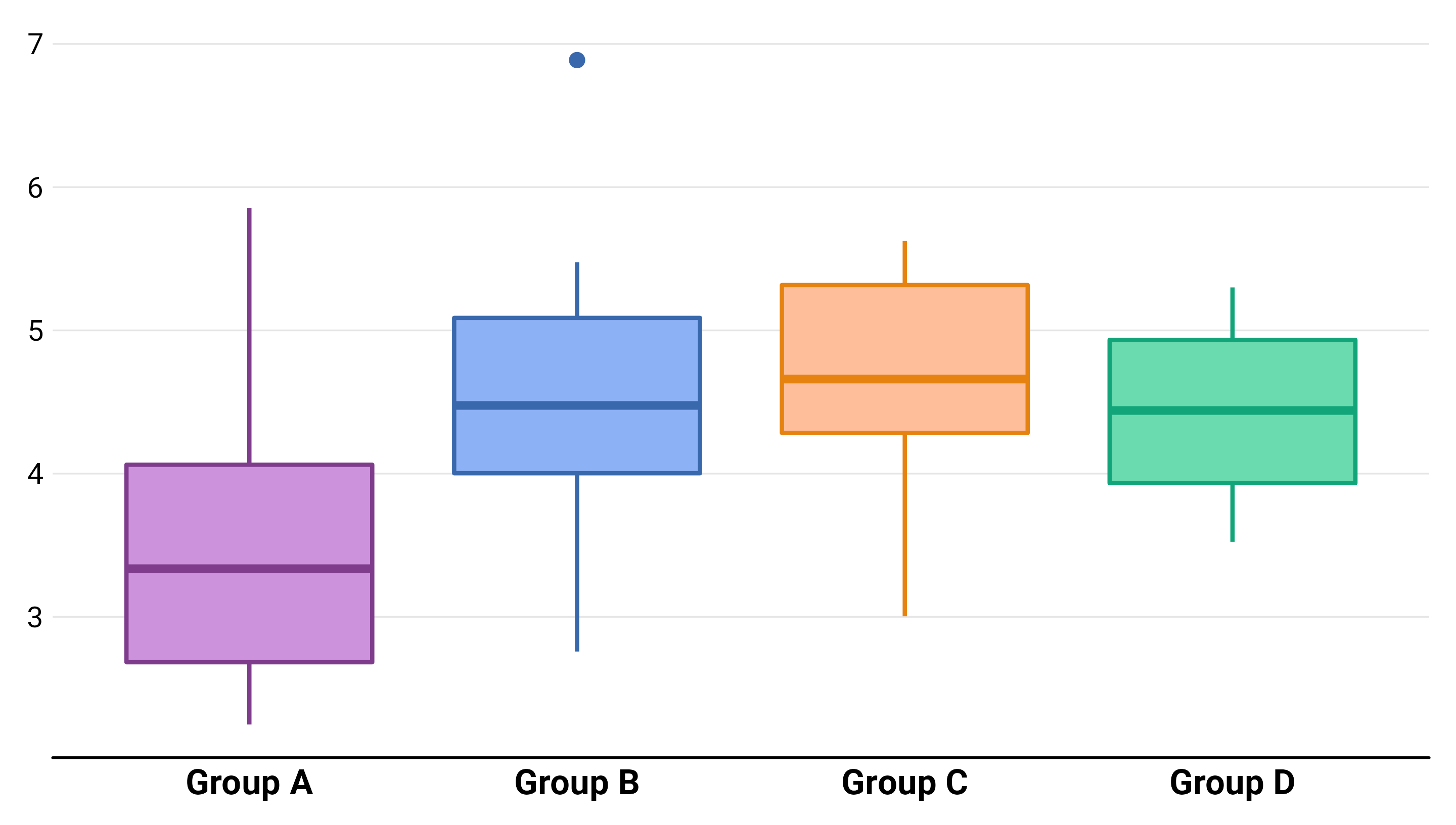While box plots are highly effective and widely used in data analytics, they are limited in the fact that they only show specific statistical points, such as the median average or outliers, rather than the distribution of a data set as a whole. ~ LondonSoda.com

### Violin Plot

Violin plots focus on illustrating the distribution of the entire data set and can generate different insights, that are hidden in the structure of box plots. ~ LondonSoda.com

``````g +
geom_violin(
aes(fill = group, fill = after_scale(colorspace::lighten(fill, .5))),
size = 1.2
)
``````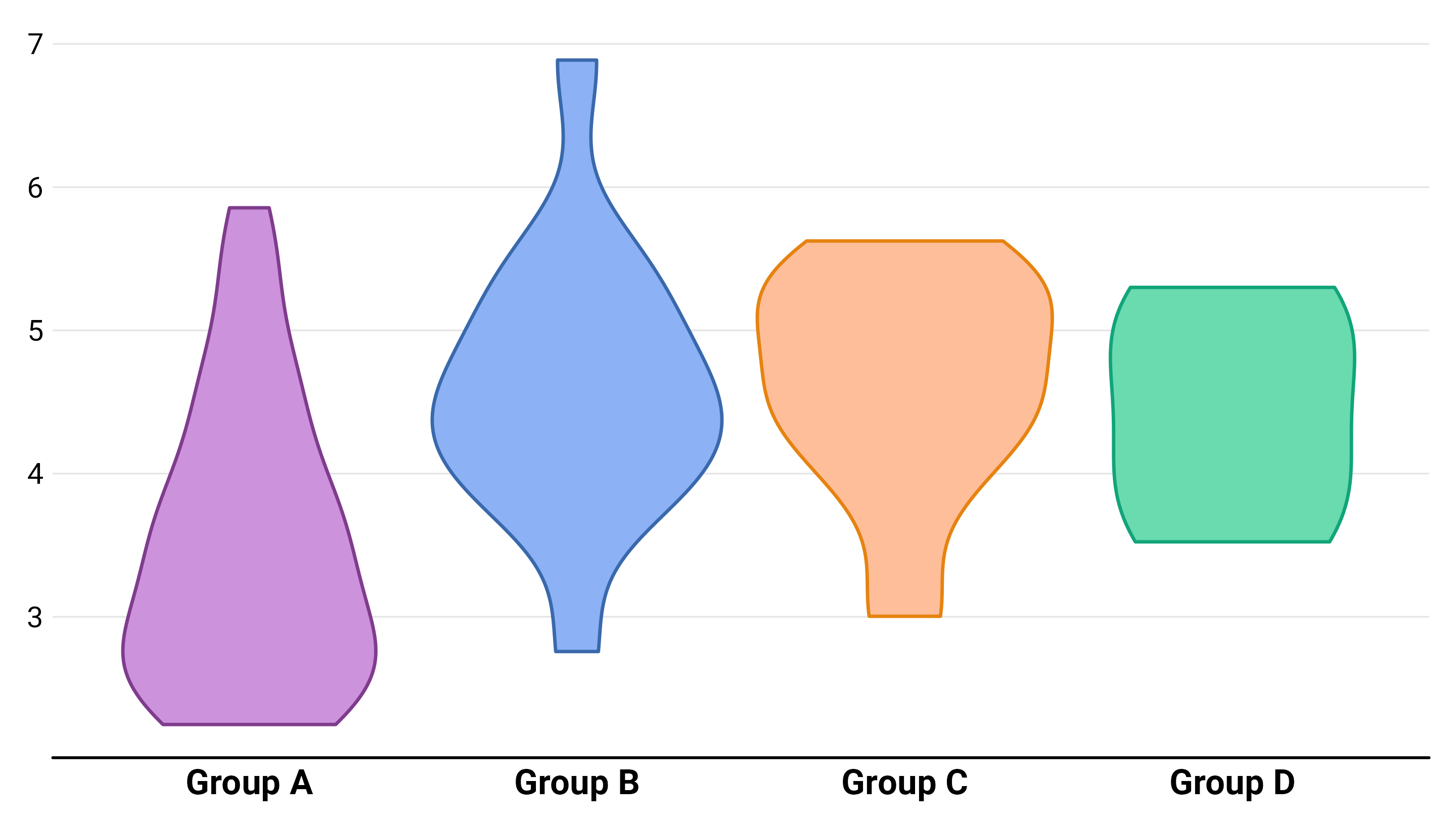``````g +
geom_violin(
aes(fill = group, fill = after_scale(colorspace::lighten(fill, .5))),
size = 1.2, bw = .2
)
``````